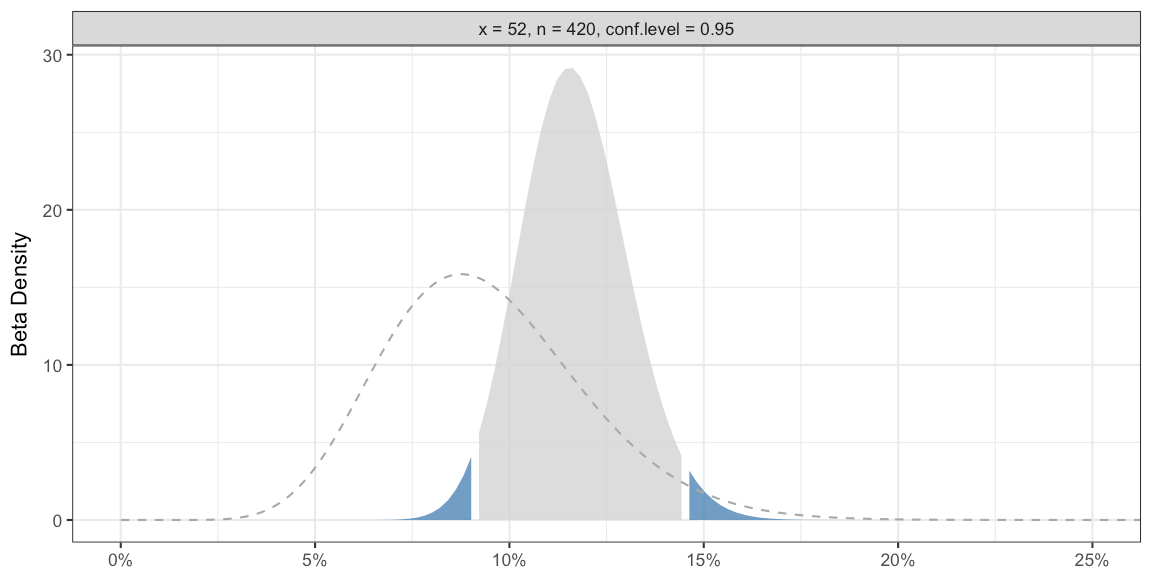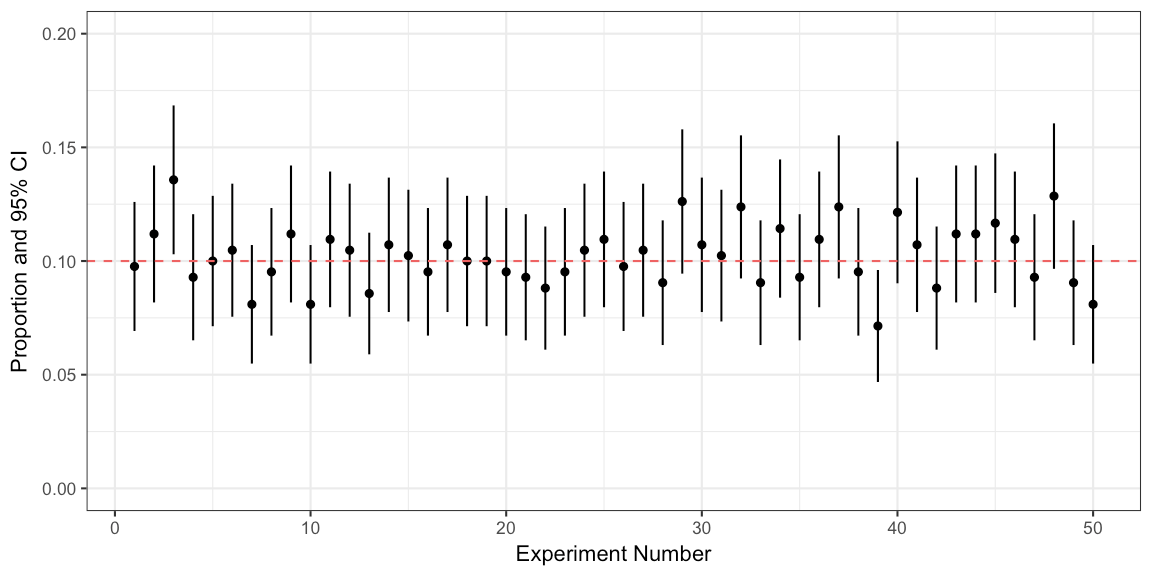Want to share your content on R-bloggers? click here if you have a blog, or here if you don't.

## Abstract

We provide an interpretation for the confidence interval for a binomial proportion hidden as the transcript of an hypothetical statistical consulting session.## The Statistical Consulting Session

[Client]: So I did this important experiment where I investigated 420 turtles whether they had a specific phenotype trait. 52 out of my 420 turtles had the trait, i.e. the proportion in the sample was 12.4%. Now I’d like to state a 95% confidence interval for what the proportion of the trait is in the population of turtles (which is pretty large).

[Statistician]: Ok, that should be possible. Since your population is large, we do not need a finite population correction, Since your sample is also pretty large, let’s therefore just go with the textbook large-sample confidence interval for a binomial proportion, that is $$\hat{p} \pm 1.96 \sqrt{\hat{p}(1-\hat{p})/n}$$. Don’t worry about the equation, let’s just use R and the binom package to compute the interval:

(ci <- binom::binom.asymp(x=x, n=n, conf.level=0.95))

##       method  x   n      mean      lower     upper
## 1 asymptotic 52 420 0.1238095 0.09231031 0.1553087

So the requested 95% CI is 9.2%- 15.5%. Happy?

[Client]: Well, yes, that looks nice, but I wonder how I can interpret the confidence interval. Is it correct to say that the interval denotes a region, which contains the true value with 95% probability?

[Statistician]: A confidence interval constructing procedure yields a random interval, because it depends on quantities (in particular $$x$$), which are random. However, once you plug-in the realization of the random variable (i.e. your observation), you get a realization of the confidence interval consisting of two numbers. Would we know the true value then we could tell, whether the value is covered by this particular interval or not. The specific interval we calculated above thus contains the true value with probability 0% or 100% - which of the two is the cases we unfortunately do not know.

The correct interpretation is thus that the confidence interval is constructed by a procedure, which, when you repeat the experiment many many times, is such that in 95% of the experiments the corresponding confidence interval would cover the true value. I can illustrate this using a small simulation study in R. Let's assume your true proportion is 10%.

##Assumed true value of the proportion of turtles with the trait
theta_true <- 0.1

##Prepare data.frame with 10000 realizations of the experiment
df <- data.frame(x=rbinom(n=1e4, size=n, prob=theta_true))

##Compute 95% CI for each experiment
df %<>% do( {
as.data.frame(cbind(.,rate=.$x/n,binom::binom.asymp(x=.$x, n=n)[c("lower","upper")]))
})
##Check if the interval covers the true value
df %<>% mutate(covers_true_value = lower < theta_true & upper > theta_true)

##Proportion of intervals covering the true value. This would be 95%
df %>% summarise(coverage = mean(covers_true_value))

##   coverage
## 1   0.9446

A graphic for the first 50 experiments shows their point estimates, corresponding intervals and how they overlap the true value or not:The specific confidence interval ci we computed above is thus just one of many possible confidence intervals originating from above procedure.

[Client]: Ok, I get that is what happens when you do it many times. But I just have this one experiment with x = 52 out of n = 420 subjects having the trait of interest. Your above output does contain a very specific CI, with some very specific numbers, i.e. 9.2%- 15.5%. Is the true value in this interval with 95% probability?

[Statistician]: No, the realized interval either covers the true value or not. But since we do not know the true value it's a bit like Schrödinger's cat... To keep things very sketchy: The width of the interval gives you an indication of your estimation certainty, but the particular values are hard to interpret - except maybe as the critical limits in a corresponding hypothesis test.

[Client]: I'm sorry, but this is useless! You suggest to follow a common statistical protocol, you compute some numbers, but these numbers don't really mean anything?

[Statistician]: Sorry, that's the way it is. However, allow me to offer a different explanation: We both appear to agree that there is a true value of the proportion, right? Let's denote this $$\theta$$ with $$0 \leq \theta \leq 1$$. We don't know the true value, but we might have some prior idea about the range of plausible values for it. Would you be willing to characterize your belief about this by a distribution for $$\theta$$? This could be something as simple as just assuming $$\theta \sim U(0,1)$$, i.e. you would assume that every value of $$\theta$$ between zero and one is equally probable initially. Then the interval we computed above denotes a 95% equal tail probability credibility region in a Bayesian framework when we assume this flat prior and use an asymptotic argument resulting in the posterior being Gaussian with the same mean and variance as the one would get from the asymptotic frequentist procedure.

[Client]: Umm, ok, but how's that helpful?

[Statistician]: In the Bayesian context a 95% credibility region is a summary of your posterior belief about the parameter. Hence, it is ok to interpret this interval as that your belief after seeing the data is such that the true value is in that interval with 95% probability.

[Client]: I love this Bayes thing! This is what I wanted initially. But hang on, I don't really believe in all values of the parameter being initially equally probable. I've seen some previous studies in the literature who under pretty similar conditions find that proportion is around 5-15%. How would I incorporate that?

[Statistician]: You could modify the large-sample Gaussian posterior such that it includes this prior information. Instead of using Gaussians you could alternatively also use a Beta distribution to perform so called conjugate prior-posterior updating of your belief about the true proportion. Let's say the 5-15% denotes your prior 95% credibility region for the parameter. We would use this to find the parameters of a beta distribution. Then we would update your prior belief with the observed data to get the posterior distribution. A feature of such a conjugate approach is that this updated distribution, i.e. the posterior, is again beta. Doing this in R is easy:

##Function to determine beta parameters s.t. the 2.5% and 97.5% quantile match the specified values
target <- function(theta, prior_interval, alpha=0.05) {
sum( (qbeta(c(alpha/2, 1-alpha/2), theta, theta) - prior_interval)^2)
}

##Find the prior parameters
prior_params <- optim(c(10,10),target, prior_interval=c(0.05, 0.15))\$par
prior_params

##   12.04737 116.06022

Actually, you can interpret the above prior parameters as the number of turtles you have seen with the trait (12) and without the trait (116), respectively, before conducting your above investigation. The posterior parameters are then just 12 + 52 = 64 turtles with the trait and 116 + 368 = 484 turtles without the trait. You get the credible interval based on this posterior in R by:

##Compute credibile interval from a beta-binomial conjugate prior-posterior approach
(ci_bayes <- binom::binom.bayes(x=x, n=n, type="central", prior.shape1=prior_params, prior.shape2=prior_params))

##   method  x   n   shape1   shape2      mean      lower     upper  sig
## 1  bayes 52 420 64.04737 484.0602 0.1168518 0.09134069 0.1450096 0.05

Graphically, your belief updating looks as follows:

##Plot of the beta-posterior
p2 <- binom::binom.bayes.densityplot(ci_bayes)
df <- data.frame(x=seq(0,1,length=1000)) %>% mutate(pdf=dbeta(x, prior_params, prior_params))
p2 + geom_line(data=df, aes(x=x, y=pdf), col="darkgray",lty=2) +
coord_cartesian(xlim=c(0,0.25)) + scale_x_continuous(labels=scales::percent)The dashed line in the graphic shows the density of your beta prior distribution. The shaded region shows the density of your beta posterior distribution, in particular, the gray shaded area denotes your 95% credibility region on the x-axis. One advantage of the beta-binomial approach is that this ensures that the prior as well as the posterior distribution have the same support as your parameter, i.e. the interval between zero and one.

[Client]: So I can really write that the interval 9.1%- 14.5% contains the true parameter with a probability of 95%?

[Statistician]: Depending on the technical level of your readership you might want to state that it's a 95% equi-tailed credible interval resulting from a beta-binomial conjugate Bayesian approach obtained when using a prior beta with parameters such that the similar 95% equi-tailed prior credible interval has limits 0.05 and 0.15. Given these assumptions the interval 9.1%- 14.5% contains 95% of your subjective posterior density for the parameter.

[Client]: ???

[Statistician]: You might just want to skip all the details and just write that the 95% Bayesian confidence interval is 9.1%- 14.5%.

[Client]: And what if the reviewers do not agree with my prior distribution?

[Statistician]: Then return to your flat prior, i.e. the uniform. Nobody usually questions that even though you just told me that you don't believe in it yourself:

(ci_bayes_unif <- binom::binom.bayes(x=x, n=n, type="central", prior.shape1=1, prior.shape2=1))

##   method  x   n shape1 shape2      mean      lower    upper  sig
## 1  bayes 52 420     53    369 0.1255924 0.09574062 0.158803 0.05

[Client]: Alright, that's settled then - I just use a Bayesian confidence interval with a flat prior and am done. That was not so hard after all. Now to something else, the reviewers of the paper, which by the way has to be revised by tomorrow, also asked us to substantiate our findings with a p-value. How would I get a p-value related to the above?

[Statistician]: Look how late it already is! I really need to go. I have this Bayesian Methods class to teach...